# Chocolates

How many ways can we distribute 8 different chocolates to four children?

n =  1680

### Step-by-step explanation: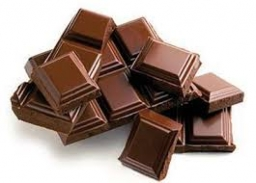Did you find an error or inaccuracy? Feel free to write us. Thank you!Tips to related online calculators
Would you like to compute count of combinations?

## Related math problems and questions:

• Chocolates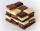In the market we have 3 kinds of chocolates. How many ways can we buy 14 chocolates?
• Chess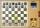How many different ways can initiate a game of chess (first pass)?
• Big numbersHow many natural numbers less than 10 to the sixth can be written in numbers: a) 9.8.7 b) 9.8.0
• Bookshelf and books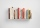How many ways can we place 7 books on a bookshelf?
• Gold, silver, bronzeHow many ways can we divide gold, silver, bronze medals if 6 people are competing?
• Coffe cups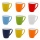We have 4 cups with 4 different patterns. How many possible combinations can we create from 4 cups?
• Possible combinations - word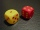How many ways can the letters F, A, I, R be arranged?
• Boys and girlsThere are 20 boys and 10 girls in the class. How many different dance pairs can we make of them?
• Toys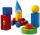3 children pulled 12 different toys from a box. Many ways can be divided toys so that each child had at least one toy?
• Guests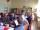How many ways can 9 guests sit down on 10 seats standing in a row?
• Math logicThere are 20 children in the group, each two children have a different name. Alena and John are among them. How many ways can we choose 8 children to be among the selected A) was John B) was John and Alena C) at least one was Alena, John D) maximum one wa
• Practice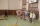How many ways can you place 20 pupils in a row when starting on practice?
• Kids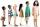How many different ways can sit 8 boys and 3 girls in line if girls want to sit on the edge?
• Bits, bytes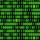Calculate how many different numbers can be encoded in 16-bit binary word?
• Shelf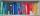How many ways are there to arrange 6 books on a shelf?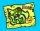There are 4 roads from city A to city B. There are 5 roads from city B to city C. How many different routes can we come from city A to city C via city B?I have 4 sweaters two are white, 1 red and 1 green. How many ways can this done?